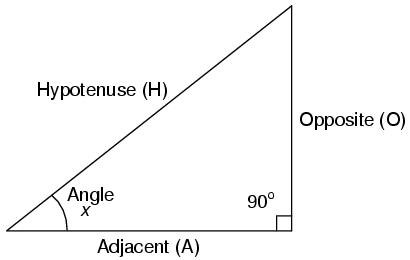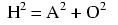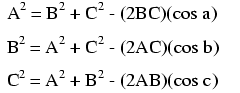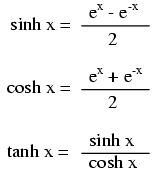# TRIGONOMETRY

### >Right triangle trigonometryA right triangle is defined as having one angle precisely equal to 90o (a right angle).

### Trigonometric identitiesH is the Hypotenuse, always being opposite the right angle. Relative to angle x, O is the Opposite and A is the Adjacent.

“Arc” functions such as “arcsin”, “arccos”, and “arctan” are the complements of normal trigonometric functions. These functions return an angle for a ratio input. For example, if the tangent of 45o is equal to 1, then the “arctangent” (arctan) of 1 is 45o. “Arc” functions are useful for finding angles in a right triangle if the side lengths are known.

### The Pythagorean theorem### Non-right triangle trigonometry### The Law of Sines (for any triangle)### The Law of Cosines## Trigonometric equivalencies## Hyperbolic functionsNote: all angles (x) must be expressed in units of radians for these hyperbolic functions. There are 2π radians in a circle (360o).

Related

COMMENT songs

1. Nidia

Solution:Step1First replace x with (x-Δx) in the given fictnuon f(x) to obtainf(x+Δx) = 2(x+Δx)+1 = 2x + 2Δx + 1 Step2Rewrite f(x-Δx) as (2x + 2Δx + 1) and f(x) as (2x + 1) into the definition of the derivative limit to obtain: lim [(2x + 2Δx + 1) – (2x+1)] / ΔxΔx-> 0 Step 3Combine like terms on the numerator and the reduced expression becomes lim [2Δx] / ΔxΔx-> 0 Step 4Cancel out Δx from both numerator and denominator and finally you get the derivative Derivative of f (x) = 2x+1 = 2 -Note there was no need to substitute Δx with 0 since it was canceled out.